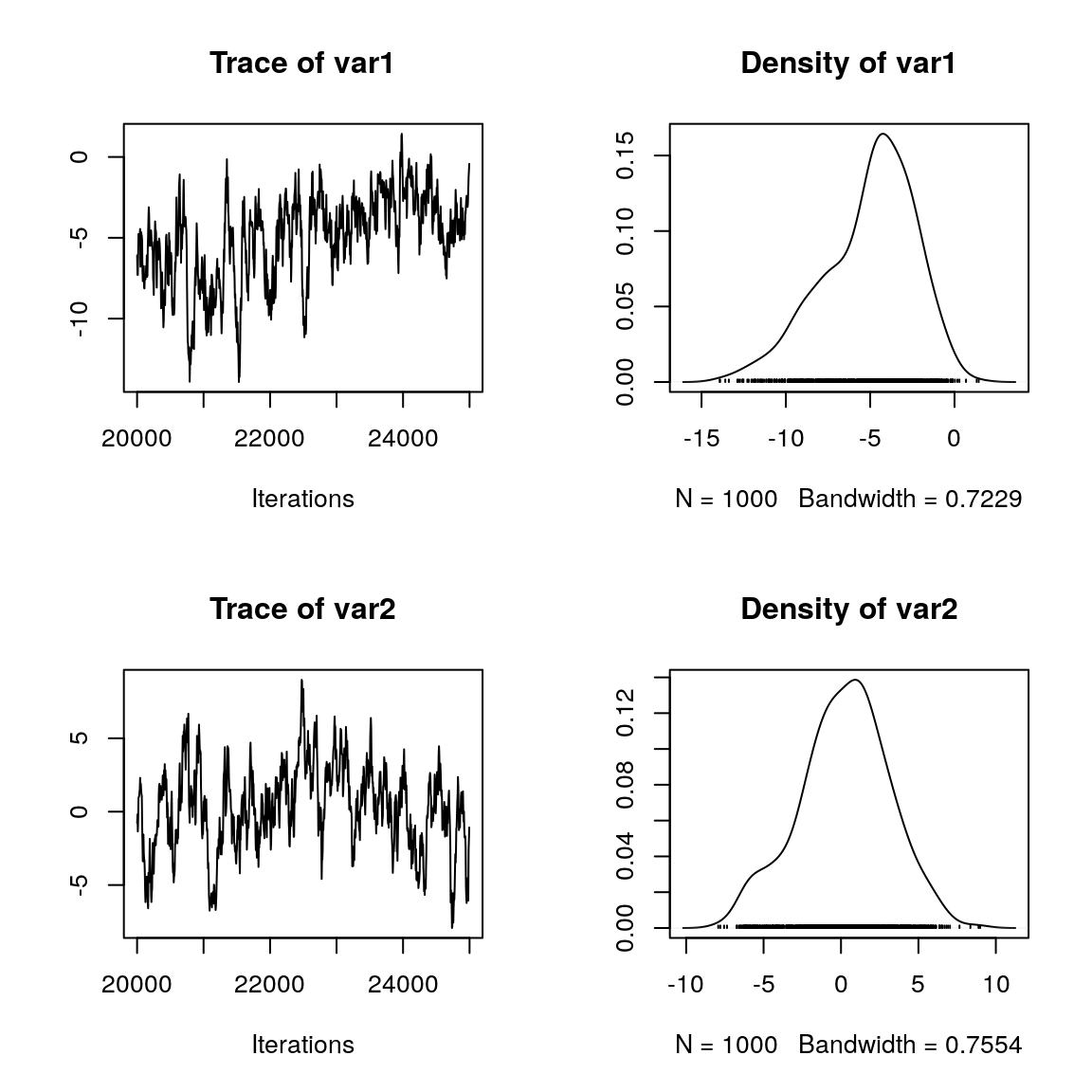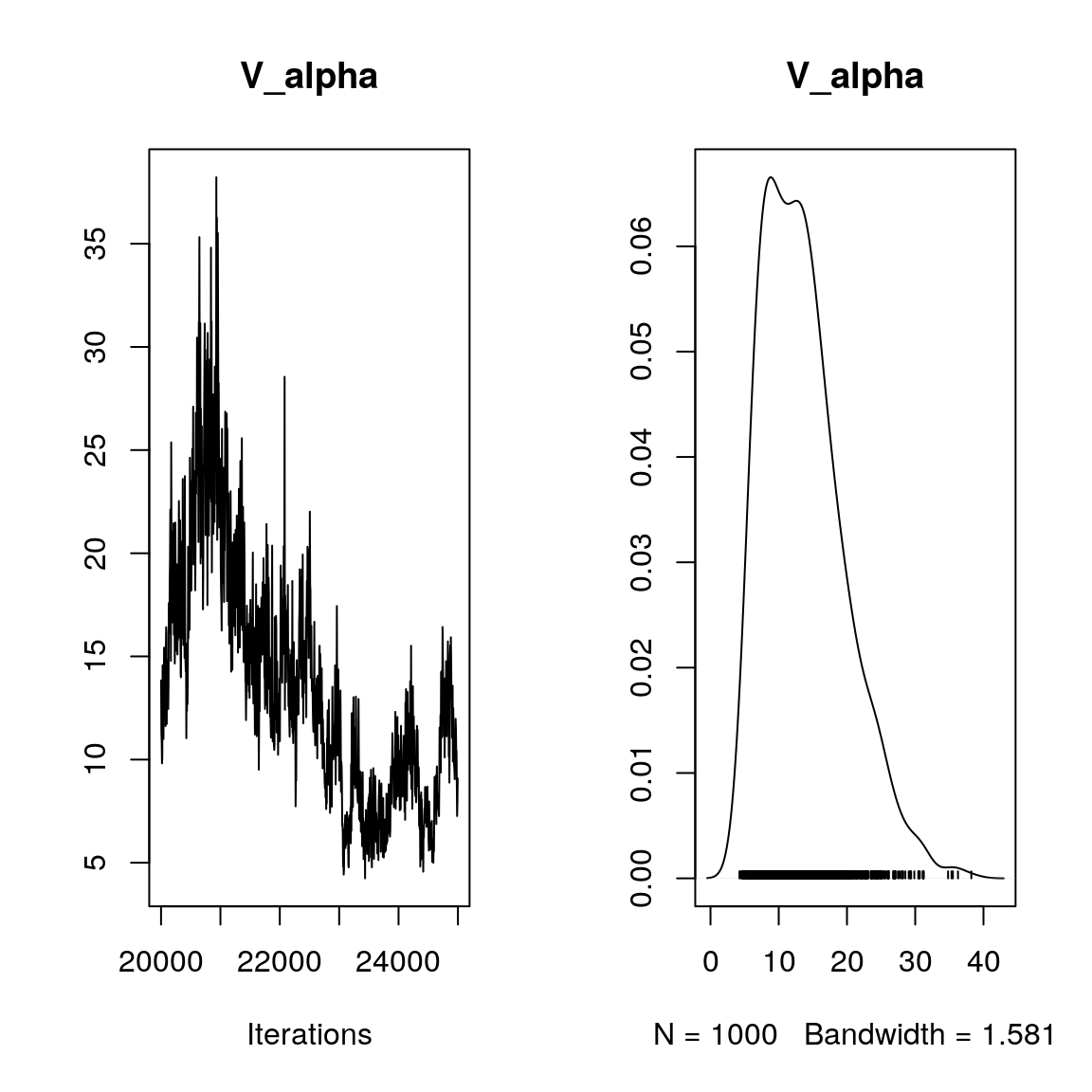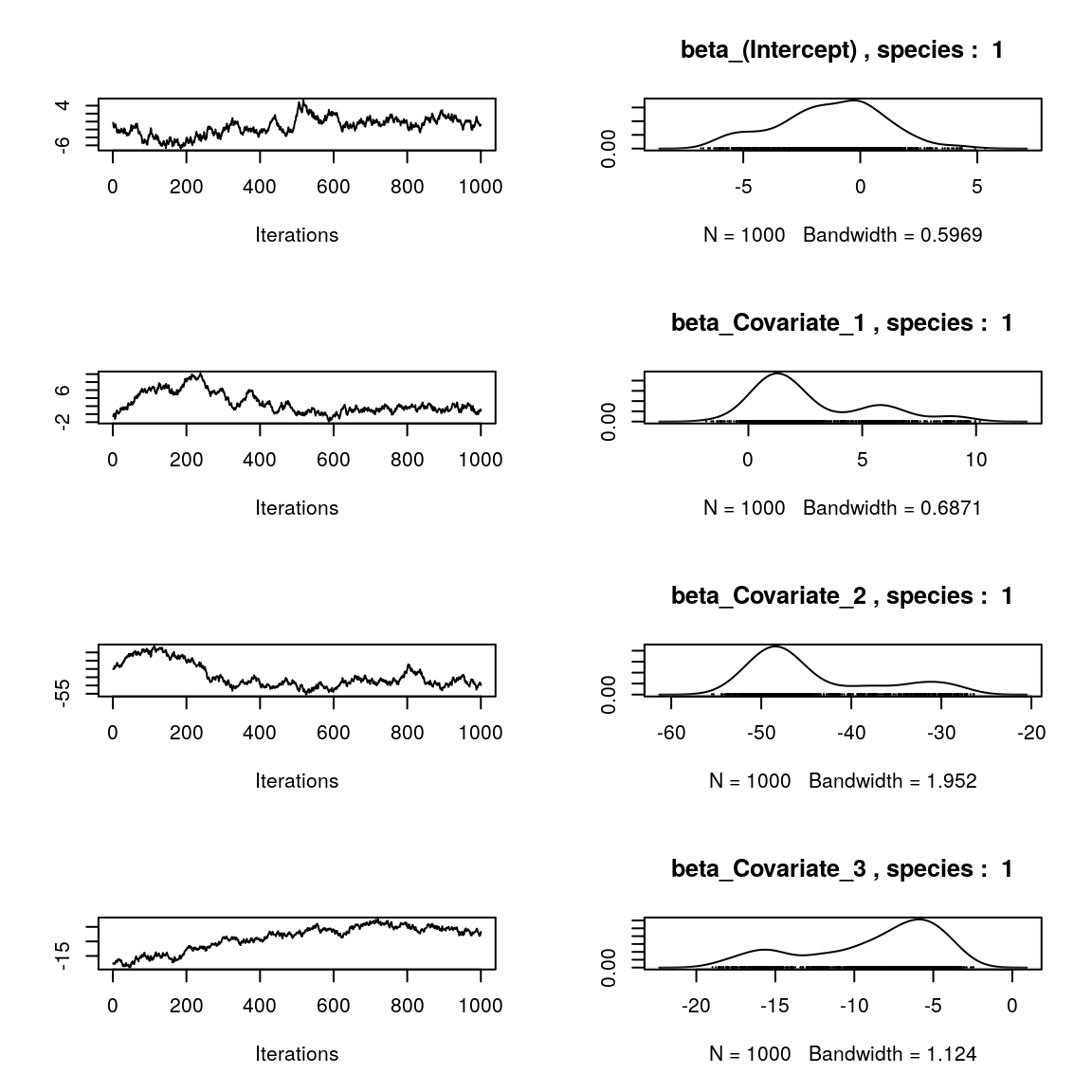Joint Species distribution models (jSDM) are useful tools to explain or predict species range and abundance from various environmental factors and species correlations (Warton et al. 2015). jSDM is becoming an increasingly popular statistical method in conservation biology. In this vignette, we illustrate the use of the jSDM R package wich aims at providing user-friendly statistical functions using field observations (occurence or abundance data) to fit jSDMs models. Package’s functions are developped in a hierarchical Bayesian framework and use conjugate priors to estimate model’s parameters. Using compiled C++ code for the Gibbs sampler reduce drastically the computation time. By making these new statistical tools available to the scientific community, we hope to democratize the use of more complex, but more realistic, statistical models for increasing knowledge in ecology and conserving biodiversity.

Below, we show an example of the use of jSDM for fitting species distribution model to abundance data for 9 frog’s species. Model types available in jSDM are not limited to those described in this example. jSDM includes various model types for occurrence data:

# 1 Definition of the model

Referring to the models used in the articles Warton et al. (2015) and Albert & Siddhartha (1993), we define the following model :

$\mathrm{probit}(\theta_{ij}) =\alpha_i + \beta_{0j}+X_i'\beta_j+ W_i'\lambda_j$

• Link function probit: $$\mathrm{probit}: q \rightarrow \Phi^{-1}(q)$$ where $$\Phi$$ correspond to the repartition function of the reduced centred normal distribution.

• Response variable: $$Y=(y_{ij})^{i=1,\ldots,nsite}_{j=1,\ldots,nsp}$$ with:

$y_{ij}=\begin{cases} 0 & \text{ if species j is absent on the site i}\\ 1 & \text{ if species j is present on the site i}. \end{cases}$

• Latent variable $$z_{ij} = \alpha_i + \beta_{0j} + X_i'\beta_j + W_i'\lambda_j + \epsilon_{i,j}$$, with $$\forall (i,j) \ \epsilon_{ij} \sim \mathcal{N}(0,1)$$ and such that:

$y_{ij}=\begin{cases} 1 & \text{if} \ z_{ij} > 0 \\ 0 & \text{otherwise.} \end{cases}$

It can be easily shown that: $$y_{ij} \sim \mathcal{B}ernouilli(\theta_{ij})$$.

• Latent variables: $$W_i=(W_i^1,\ldots,W_i^q)$$ where $$q$$ is the number of latent variables considered, which has to be fixed by the user (by default q=2). We assume that $$W_i \sim \mathcal{N}(0,I_q)$$ and we define the associated coefficients: $$\lambda_j=(\lambda_j^1,\ldots, \lambda_j^q)'$$. We use a prior distribution $$\mathcal{N}(0,10)$$ for all lambdas not concerned by constraints.

• Explanatory variables: bioclimatic data about each site. $$X=(X_i)_{i=1,\ldots,nsite}$$ avec $$X_i=(x_i^1,\ldots,x_i^p)'\in \mathbb{R}^p$$ where $$p$$ is the number of bioclimatic variables considered. The corresponding regression coefficients for each species $$j$$ are noted : $$\beta_j=(\beta_j^1,\ldots,\beta_j^p)'$$.

• $$\beta_{0j}$$ correspond to the intercept for species $$j$$ which is assume to be a fixed effect. We use a prior distribution $$\mathcal{N}(0,10^6)$$ for all betas.

• $$\alpha_i$$ represents the random effect of site $$i$$ such as $$\alpha_i \sim \mathcal{N}(0,V_{\alpha})$$ and we assumed that $$V_{\alpha} \sim \mathcal {IG}(\text{shape}=0.5, \text{rate}=0.005)$$ as prior distribution by default.

# 2 Data-setFigure 2.1: Litoria ewingii (Wilkinson et al. 2019).

We first load the jSDM library.

# Load libraries
library(jSDM)
#> ##
#> ## jSDM R package
#> ## For joint species distribution models
#> ## https://ecology.ghislainv.fr/jSDM
#> ##

This data-set is available in the jSDM R package. It can be loaded with the data command. The data is in “wide” format: each line is a site and the occurrence data (from Species_1 to Species_9) are in columns. A site is characterized by its x-y geographical coordinates, one discrete covariate and two other continuous covariates.

# frogs data
data(frogs, package="jSDM")
#>   Covariate_1 Covariate_2 Covariate_3 Species_1 Species_2 Species_3
#> 1    3.870111           0    0.045334         1         0         0
#> 2    3.326950           1    0.115903         0         0         0
#> 3    2.856729           1    0.147034         0         0         0
#> 4    1.623249           1    0.124283         0         0         0
#> 5    4.629685           1    0.081655         0         0         0
#> 6    0.698970           1    0.107048         0         0         0
#>   Species_4 Species_5 Species_6 Species_7 Species_8 Species_9        y
#> 1         0         0         0         0         0         0 66.41479
#> 2         0         0         1         0         0         0 67.03841
#> 3         0         0         1         0         0         0 67.03855
#> 4         0         0         1         0         0         0 67.04200
#> 5         0         0         1         0         0         0 67.04439
#> 6         0         0         0         0         0         0 67.03894
#>          x
#> 1 9.256424
#> 2 9.025588
#> 3 9.029416
#> 4 9.029745
#> 5 9.026514
#> 6 9.023580

We rearrange the data in two data-sets: a first one for the presence-absence observations for each species (columns) at each site (rows), and a second one for the site characteristics.

We also normalize the continuous explicative variables to facilitate MCMC convergence.

# data.obs
PA_frogs <- frogs[,4:12]

# Normalized continuous variables
Env_frogs <- cbind(scale(frogs[,1]),frogs[,2],scale(frogs[,3]))
colnames(Env_frogs) <- colnames(frogs[,1:3])

# 3 Parameter inference

We use the jSDM_probit_block() function.

mod_jSDM_block_frogs <- jSDM_probit_block (
# Response variable
presence_site_sp = as.matrix(PA_frogs),
# Explanatory variables
site_suitability = ~.,
site_data = as.data.frame(Env_frogs), n_latent=2,
# Chains
burnin=20000, mcmc=5000, thin=5,
# Starting values
alpha_start=0, beta_start=0,
lambda_start=0, W_start=0,
V_alpha_start=1,
# Priors
shape=0.5, rate=0.0005,
mu_beta=0, V_beta=1.0E6,
mu_lambda=0, V_lambda=10,
# Various
seed=1234, verbose=1)
#>
#> Running the Gibbs sampler. It may be long, please keep cool :)
#>
#> **********:10.0%
#> **********:20.0%
#> **********:30.0%
#> **********:40.0%
#> **********:50.0%
#> **********:60.0%
#> **********:70.0%
#> **********:80.0%
#> **********:90.0%
#> **********:100.0%

# 4 Analysis of the results

## alpha_i of the first two sites
plot(coda::as.mcmc(mod_jSDM_block_frogs$mcmc.alpha[,1:2]))## Valpha par(mfrow=c(1,2)) coda::traceplot(mod_jSDM_block_frogs$mcmc.Valpha, main="V_alpha")
coda::densplot(mod_jSDM_block_frogs$mcmc.Valpha, main="V_alpha")np <- nrow(mod_jSDM_block_frogs$model_spec$beta_start) ## beta_j of the first two species par(mfrow=c(np,2)) for (j in 1:2) { for (p in 1:np) { coda::traceplot(coda::as.mcmc(mod_jSDM_block_frogs$mcmc.sp[[paste0("sp_",j)]][,p]))
coda::densplot(coda::as.mcmc(mod_jSDM_block_frogs$mcmc.sp[[paste0("sp_",j)]][,p]), main = paste(colnames(mod_jSDM_block_frogs$mcmc.sp[[paste0("sp_",j)]])[p],
", species : ",j))
}
}